### IMO Shortlist 1974 problem 5

Kvaliteta:
Avg: 0,0
Težina:
Avg: 0,0
Let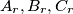$A_r,B_r, C_r$ be points on the circumference of a given circle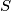$S$. From the triangle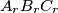$A_rB_rC_r$, called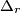$\Delta_r$, the triangle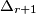$\Delta_{r+1}$ is obtained by constructing the points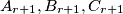$A_{r+1},B_{r+1}, C_{r+1}$on$S$ such that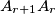$A_{r+1}A_r$ is parallel to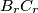$B_rC_r$,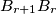$B_{r+1}B_r$ is parallel to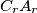$C_rA_r$, and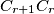$C_{r+1}C_r$ is parallel to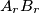$A_rB_r$. Each angle of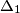$\Delta_1$ is an integer number of degrees and those integers are not multiples of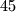$45$. Prove that at least two of the triangles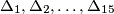$\Delta_1,\Delta_2, \ldots ,\Delta_{15}$ are congruent.
Izvor: Međunarodna matematička olimpijada, shortlist 1974